# divide and conquer

theme
• take Θ( f(n) ) runtime algorithm

• divide into k subproblems

• recombine with Θ( g(n) ) method, where g(n) = o(f(n))

• result: o( f(n) ) algorithm

multiplication   recurrence relations   sort/search   sorting lower bound

## multiplication

Gauss observation
```(a  bi)(c  di) =
(ac-bd)  (bc + ad)i   4 mult, 2 add/sub

x: ac   y: bd   z: (a+b)(c+d)

(a  bi)(c  di) =
(x-y)  (z-x-y)i       3 mult, 5 add/sub
```
Karatsuba d&c multiplication
```def bitlength(n): return len(bin(n))-2

def leftright(x,n): # x > 1
left  = x >> n/2
right = x - (left << n/2)
return left, right

def fastmul(x,y): # x,y > 0
n = bitlength(x)
if n <= 4:
return x*y
xl, xr = leftright(x,n)
yl, yr = leftright(y,n)
pl = fastmul(xl,yl)
pr = fastmul(xr,yr)
pm = fastmul(xl+xr,yl+yr)
return (pl << 2*(n/2)) + ((pm - pl - pr) << n/2) + pr

for j in range(400):
for k in range(400):
assert( fastmul(j,k) == j*k)
```
Karatsuba correctness example
```- assume 7 digit inputs
- shift <-  7/2 = 3
- split inputs:  aaaa bbb    cccc ddd
-                 a'   b'     c'   d'
- L <- a' * c'
- R <- b' * d'
- S <- (a' + b') * (c' + d')

- return     L * base^(2*shift)  +
(S-L-R) * base^shift   +
R
- correct ?
aaaa bbb
*  cccc ddd
--------
llllllll  mmmmmmm  rrrrrr
a'c'   a'd'+b'c'   b'd'

- want L = a'c'                check :)
- want R = b'd'                check :)
- want M = S-L-R = b'c' + a'd'       ???
S-L-R = a'c' + b'c' + a'd' + b'd - a'c' - b'd'
=        b'c' + a'd'     check :)
```
Karatsuba decimal example
```return x*y if x <= 10 or y <= 10
(1234,5678)
(12,56)
(1,5) 5
(2,6) 12
1+2,5+6
(3,11) 33
5  33-5-12  12
500 + 160 + 12
672
(34,78)
(3,7) 21
(4,8) 32
3+4,7+8
(7,15) 105
21 105-21-32 32
2100 + 520 + 32
2652
12+34,56+78
(46,134)
(4,13) 52
(6,4)  24
4+6,13+4
(10,17) 170
52 170-52-24 24
5200 + 940 + 24
6164
672  6164-672-2652  2652
6720000 + 284000 + 2652
7006652
```
runtime
• T(n) = 3T(n/2) + O(n)

• will see T(n) = O(nlg 3) = O(n1.58..)

can we do better ?
• O(n2)       school, peasant

• O(nlg 3)       Karatsuba

• O(nlog35)       Toom-Cook 3-way

• O(nlog n log log n)       Schonhage-Strassen

• O(nlog n 2O(log * n))       Furer

• in practice, faster algorithms not worth it unless n large

• python uses school for small n, Karatsuba for large n

## recurrence relations

solving a simple recurrence relation
master theorem

integers a>0, b>1, real d≥0, T(n) = a T(n/b) + O(nd),

• if bd > a     ( d > lgb a )   then     T(n) ∈ O(nd)

• if bd = a     ( d = lgb a )   then     T(n) ∈ O(nd lg n)

• if bd < a     ( d < lgb a )   then     T(n) ∈ O(nlgba)

proof (special case)
• assume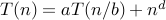for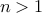• assume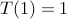• assume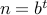•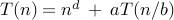•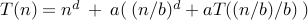•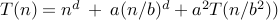•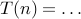•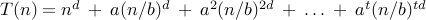•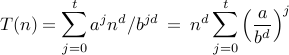• let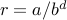• if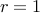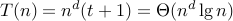• if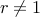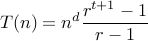• if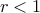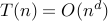• if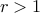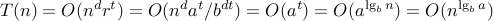example
• T(n) = 3 T(n/2) + O(n)

• a=3 b=2 d=1   T(n) ∈ O(nlg 3) = O(n1.58..)

## search/sort

sorting and search examples
• key     a list item

• usual performance measure: count key comparisons

• e.g. binary search, key comparisons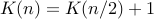• master theorem: a=1, b=2, d=0, so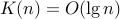remark
• to accurately measure binary search runtime, need to know

• how big are numbers in list ?

• how is division by 2 implemented ?

• how is key comparison implemented ?

• e.g. each list item: {1,…,m}, div2: shift, compare: subtract

• then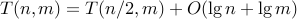mergesort
```def ms(x): # based on:
#http://stackoverflow.com/questions/18761766/mergesort-python
result = []
if len(x) < 2:
return x
mid = len(x)/2 ; y = ms(x[:mid]) ; z = ms(x[mid:])
i,j = 0,0
while i < len(y) and j < len(z):
if y[i] > z[j]:
result.append(z[j]) ; j += 1
else:
result.append(y[i]) ; i += 1
result += y[i:] + z[j:]
return result
```
randomized selection
```def select(L,k):
A = []; B = [];  C = []
v = L[random.randint(0,len(L)-1)]
for j in L:
if j<v:
A.append(j)
elif j>v:
C.append(j)
else:
B.append(j)
if k <= len(A):
return select(A,k)
if k <= len(A) + len(B):
return v
return select(C,k-len(A)-len(B))
```
runtime ?
• worst case: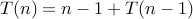so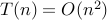• best case: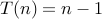• average case: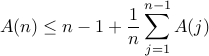• prove by induction: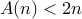, so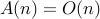partition
```# partition, qs:http://hetland.org/coding/python/quicksort.html
def partition(list, start, end):
pivot = list[end]    # partition around the last value
bottom = start-1     # initially outside sublist to be partitioned
top = end            # "         "
done = 0
while not done:      # until all elements partitioned...
while not done:  # until we find an out of place element...
bottom = bottom+1  # move the bottom up.
if bottom == top:  # if hit top...
done = 1       # ... we are done.
break
if list[bottom] > pivot:     # bottom out of place?
list[top] = list[bottom] # put it at top...
break                    # ... resume search from top
while not done:        # until we find an out of place element...
top = top-1        # move top down.
if top == bottom:  # if hit bottom...
done = 1       # ... we are done.
break
if list[top] < pivot:        # top out of place?
list[bottom] = list[top] # put it at bottom...
break                    # ... resume search from bottom
list[top] = pivot                    # replace pivot
```
quicksort
```def quicksort(list, start, end):
if start < end:        # two or more elements?
split = partition(list, start, end)
quicksort(list, start, split-1)
quicksort(list, split+1, end)
return
```
quicksort runtime
•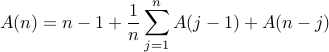• can show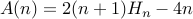, where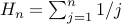• with high prob., number of key comparisons close to average

• so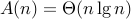• wiki

• improvements

• pick splitter to be median of L, L[n/2], L[n]

• recursively sort smaller subproblem (so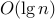stack depth), eliminate remaining tail recursion

## sorting lower bound

comparison based sorting algorithm
• only operation allowed on keys: <

• e.g. allowed       L < L ?

• e.g. not allowed       L > (L + L) ?

• consider decision tree: after 1st comparison, branch left or right

• number tree leaves must be at least number of possible inputs = n!

• after t comparisons, at most 2t tree leaves

• so for m = n! tree leaves we need at least lg (n!) comparisons

• lg (n!) ∈ Θ(n lg n)

• so any comparison-based sort of n keys takes Ω(n lg n) time

example
• can we comparison-sort 6 keys with at most 9 key comparisons?

• no       6!= 720, and 29 = 512 < 720, so lg 720 > 9